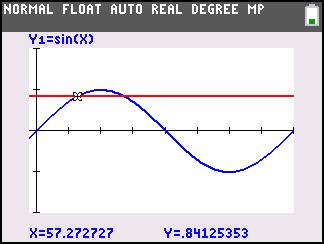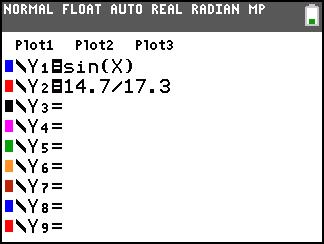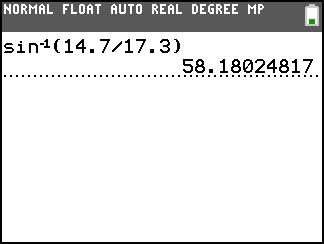••• ##### Device
• TI-83 Plus Family
• TI-84 Plus
• TI-84 Plus Silver Edition
•TI-84 Plus C Silver Edition
•TI-84 Plus CE
• ##### Report an Issue

Algebra II: Solving Trigonometric Equations
by Texas Instruments#### Overview

Students represent and analyze mathematical situations and structures using algebraic symbols.

#### Key Steps

•Students consider a triangle ABC. They use the sine ratio to determine the measure of angle A.

They use their calculator to plot the graph for the ratio and the sine.

•After graphing the two, students use TRACE to help students see that the ratio 14.7/17.3 is about 0.8497.

Students move to one of the intersection points. It appears to be about 124°. Then they move to another intersection point, it appears to be about 57°.

They will see that either 124° or 57°, is going to be the measurement of angle

•To get a more exact estimate, examine a table in smaller increments or students can by using the intersect tool.

Another choice that students have is finding the sine inverse of the ratio.

At the end of this activity, students will know several ways to find a missing angle of a right triangle. They will also be able to find the intersection of two graphs.